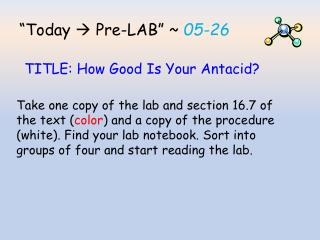DownloadDownload Presentation“Today  Pre-LAB ” ~ 05-26

# “Today  Pre-LAB ” ~ 05-26

Télécharger la présentation## “Today  Pre-LAB ” ~ 05-26

- - - - - - - - - - - - - - - - - - - - - - - - - - - E N D - - - - - - - - - - - - - - - - - - - - - - - - - - -
##### Presentation Transcript

1. “Today  Pre-LAB” ~ 05-26 TITLE: How Good Is Your Antacid? Take one copy of the lab and section 16.7 of the text (color) and a copy of the procedure (white). Find your lab notebook. Sort into groups of four and start reading the lab.

2. We will fill out the first part of the lab notebook together Title: (fill in the table of contents and start the next blank page of your lab journal) “How Good Is Your Antacid?” Purpose: Use objective #1 in the lab handout as your purpose. You will not complete objective #2. There is no hypothesis for this lab.

3. Introduction: Leave space for a simple introduction explaining the purpose of the lab and the equations. The active ingredients for Tums are (calcium carbonate, CaCO3(s) )and for Rolaids are (calcium carbonate and magnesium hydroxide, Mg(OH)2(s)) Write the balanced chemical equations for the neutralization reactions of these antacids.

4. Introduction: In this experiment a concept called back titration will be used. Excess acid is mixed with a known amount of antacid and then the remaining acid is titrated with 0.10 M NaOH to the phenolphthalein endpoint. An indicator signals the endpoint of a titration, usually by causing the solution in the receiving flask to change color.

5. Materials: Transfer the materials list from the lab handout to your lab notebook Procedure: Trim the procedure handout (white half-page) to fit your lab notebook and paste it in using a glue stick

6. Data and Observations: Draw your data table and calculation table and leave lots of room to show your calculations and your results/calculations table. • Data table column headers: • Antacid • Trial # • Whole tablet mass (g) • Sample mass (g) • Volume added 0.20 MHClmL • NaOH 0.10 MBuret Volume, Initial, mL • NaOH 0.10 MBuret Volume, Final, mL • Total volume NaOH 0.10 M added, mL

7. Data and Observations: Draw your data table and calculation table and leave lots of room to show your calculations and your results/calculations table. • Determine calculation table headers for each needed calculation: • Moles of NaOH added = moles of HCl left • Moles HCl neutralized = initial moles HCl - Moles HCl left • Moles HCl neutralized ÷ Antacid sample mass • Averages for each antacid

8. Conclusion: Restate the purpose and clearly state your results. Refer specifically to your data. Error Analysis: Be sure to state for any error the result that error has on your final calculations. Example: does is skew your results toward more effectiveness or less effectiveness of the tested antacid.

9. Calculations: The calculations needed for this lab are outlined in the handout and the section of text provided. Leave lots of space in your journal for calculations. All calculation results in your calculation table must have the corresponding calculation shown in this section Pre-lab Questions and Reading: Complete the pre-lab questions and reading before you start the lab on Wednesday or Thursday